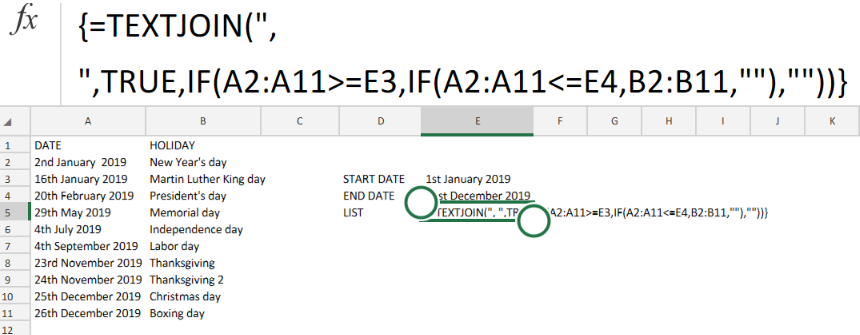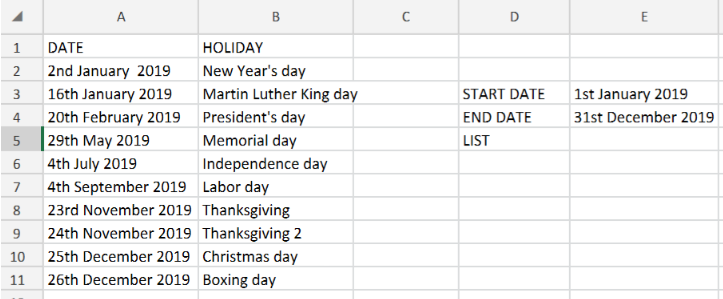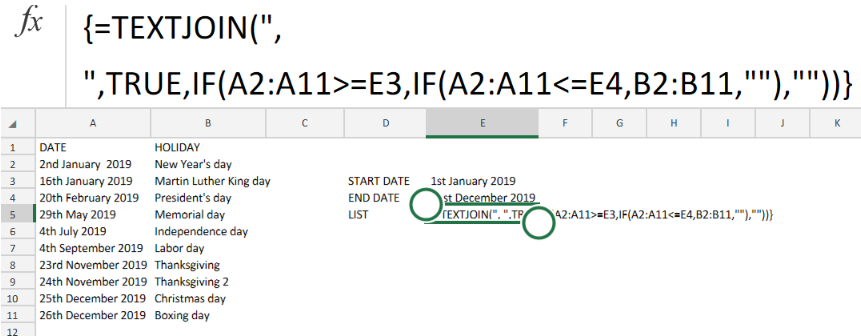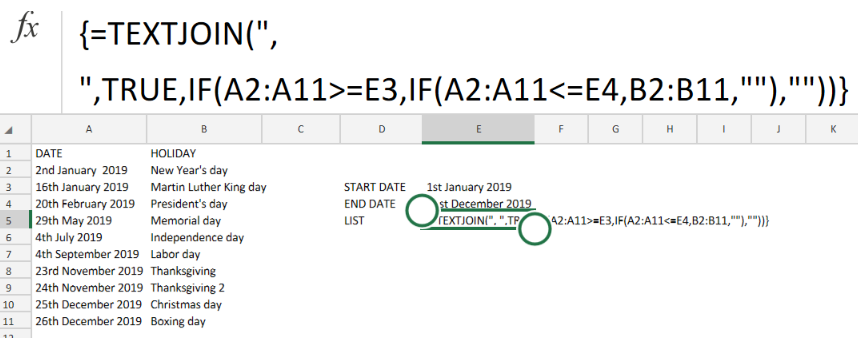Get instant live expert help with Excel or Google Sheets“My Excelchat expert helped me in less than 20 minutes, saving me what would have been 5 hours of work!”

#### Post your problem and you’ll get expert help in seconds.

Your message must be at least 40 characters
Our professional experts are available now. Your privacy is guaranteed.

# List holidays between two datesFigure 1. List Holidays Between Two Dates in Excel.

If we are required to list out every single holiday that occurs between two specific dates, we have to utilize a formula derived from the Excel IF and TEXTJOIN functions.

## Generic Formula

`{=TEXTJOIN(", ",TRUE,IF(B4:B12>=F5,IF(B4:B12<=F6,C4:C12,""),""))}`

This modified formula operates by using a series of nested IF functions to return a list of holiday dates occurring between two specific dates.

The TEXTJOIN function then proceeds to process the list of holidays and converts them into text, separated by commas.

## How to use the Excel IF and TEXTJOIN functions.

We are now going to use the Excel IF and TEXTJOIN functions to return a list of holidays between two dates in our worksheet.

We are going to achieve this by following 3 simple steps;

1. Create a list of calendar dates and public holidays in the columns of our worksheet.

See example illustrated below;Figure 2. List Holidays Between Two Dates in Excel.

Be sure to provide a blank cell for Excel to return our list of holidays (cell F5 above).

1. Enter the formula for the Excel IF and TEXTJOIN functions into the cell F5.

The formula in this case would be;

`{=TEXTJOIN(", ",TRUE,IF(A2:A11>=E3,IF(A2:A11<=E4,B2:B11,""),""))}`Figure 3. List Holidays Between Two Dates in Excel.

In this case, our specified start date is 1st of January 2019 (cell E3), while our end date is 31st December 2019 (cell E4).Figure 4. Final Result.

## Instant Connection to an Expert through our Excelchat Service:

Our live Excelchat Service is here for you. We have Excel Experts available 24/7 to answer any Excel questions you may have. Guaranteed connection within 30 seconds and a customized solution for you within 20 minutes.

### Did this post not answer your question? Get a solution from connecting with the expert.Another blog reader asked this question today on Excelchat:
Solution examplesI need a range of numbers to become the number in the next column
Solved by D. F. in 47 minsIs there an actual function that changes cell colour rather than Conditional Formatting? It wouldn't work in this situation. I basically have an IF statement and I want the TRUE result to be the colour of the cell changing. Ive tried setting it to "1", then setting a rule where all "1" become that colour, but nothing happens. I've tried it with and without the quotations.
Solved by F. J. in 28 minsCould you help me by explaining the IF function?
Solved by G. L. in 24 minsi need a formla to say if a colum is above 0% then its Marked as "Target OFF"
Solved by A. A. in 20 minsI have a question about Conditional Formatting. =IF('Sheet1'!A1="X",IF('Sheet1'!B1="Y", TRUE, FALSE),FALSE) the set the cell background to red. I would like to make it so that if Sheet1'!B1="Z" I set the back to blue. How can I achieve this.
Solved by B. F. in 39 mins## Subscribe to Excelchat.coAnother blog reader asked this question today on Excelchat: